Java集合详解4：一文读懂HashMap和HashTable的区别以及常见面试题

《Java集合详解系列》是我在完成夯实Java基础篇的系列博客后准备开始写的新系列。

https://github.com/h2pl/Java-Tutorial

www.how2playlife.com

## HashMap

HashMap也是我们使用非常多的Collection，它是基于哈希表的 Map 接口的实现，以key-value的形式存在。在HashMap中，key-value总是会当做一个整体来处理，系统会根据hash算法来来计算key-value的存储位置，我们总是可以通过key快速地存、取value。下面就来分析HashMap的存取。

### 定义

HashMap实现了Map接口，继承AbstractMap。其中Map接口定义了键映射到值的规则，而AbstractMap类提供 Map 接口的骨干实现，以最大限度地减少实现此接口所需的工作，其实AbstractMap类已经实现了Map，这里标注Map LZ觉得应该是更加清晰吧！

``````public class HashMap<K,V>
extends AbstractMap<K,V>
implements Map<K,V>, Cloneable, Serializable
``````

### 构造函数

``````  HashMap提供了三个构造函数：

HashMap()：构造一个具有默认初始容量 (16) 和默认加载因子 (0.75) 的空 HashMap。

HashMap(int initialCapacity)：构造一个带指定初始容量和默认加载因子 (0.75) 的空 HashMap。

``````

HashMap是一种支持快速存取的数据结构，要了解它的性能必须要了解它的数据结构。

HashMap数据结构图

### 存储实现：put(key,vlaue)

``````  首先我们先看源码

public V put(K key, V value) {
//当key为null，调用putForNullKey方法，保存null与table第一个位置中，这是HashMap允许为null的原因
if (key == null)
return putForNullKey(value);
//计算key的hash值，此处对原来元素的hashcode进行了再次hash
int hash = hash(key.hashCode());                  ------(1)
//计算key hash 值在 table 数组中的位置
int i = indexFor(hash, table.length);             ------(2)
//从i出开始迭代 e,找到 key 保存的位置
for (Entry<K, V> e = table[i]; e != null; e = e.next) {
Object k;
//判断该条链上是否有hash值相同的(key相同)
//若存在相同，则直接覆盖value，返回旧value
if (e.hash == hash && ((k = e.key) == key || key.equals(k))) {
V oldValue = e.value;    //旧值 = 新值
e.value = value;
e.recordAccess(this);
return oldValue;     //返回旧值
}
}
//修改次数增加1
modCount++;
//将key、value添加至i位置处
return null;
}
``````

1、 先看迭代处。此处迭代原因就是为了防止存在相同的key值，若发现两个hash值（key）相同时，HashMap的处理方式是用新value替换旧value，这里并没有处理key，这就解释了HashMap中没有两个相同的key。

2、 在看（1）、（2）处。这里是HashMap的精华所在。首先是hash方法，该方法为一个纯粹的数学计算，就是计算h的hash值。

``````static int hash(int h) {
h ^= (h >>> 20) ^ (h >>> 12);
return h ^ (h >>> 7) ^ (h >>> 4);
}
``````

``````static int indexFor(int h, int length) {
return h & (length-1);
}
``````

HashMap的底层数组长度总是2的n次方，在构造函数中存在：capacity <<= 1;这样做总是能够保证HashMap的底层数组长度为2的n次方。当length为2的n次方时，h&(length - 1)就相当于对length取模，而且速度比直接取模快得多，这是HashMap在速度上的一个优化。至于为什么是2的n次方下面解释。

==对length取模来得到hash是常用的hash索引方法，这里采用位运算的话效率更高。==

``````void addEntry(int hash, K key, V value, int bucketIndex) {
//获取bucketIndex处的Entry
Entry<K, V> e = table[bucketIndex];
//将新创建的 Entry 放入 bucketIndex 索引处，并让新的 Entry 指向原来的 Entry
table[bucketIndex] = new Entry<K, V>(hash, key, value, e);
//若HashMap中元素的个数超过极限了，则容量扩大两倍
if (size++ >= threshold)
resize(2 * table.length);
}
``````

``````后面添加的entry反而会接到前面。
``````

### JDK1.8的hashmap：put方法

``````final V putVal(int hash, K key, V value, boolean onlyIfAbsent,
boolean evict) {
Node<K,V>[] tab; Node<K,V> p; int n, i;
if ((tab = table) == null || (n = tab.length) == 0)
n = (tab = resize()).length;
if ((p = tab[i = (n - 1) & hash]) == null)
tab[i] = newNode(hash, key, value, null);
else {
Node<K,V> e; K k;
if (p.hash == hash &&
((k = p.key) == key || (key != null && key.equals(k))))
e = p;
//如果p是红黑树节点，则用另外的处理方法
else if (p instanceof TreeNode)
e = ((TreeNode<K,V>)p).putTreeVal(this, tab, hash, key, value);
else {
for (int binCount = 0; ; ++binCount) {
if ((e = p.next) == null) {
p.next = newNode(hash, key, value, null);
if (binCount >= TREEIFY_THRESHOLD - 1) // -1 for 1st
//当链表节点数超过8个，则直接进行红黑树化。
treeifyBin(tab, hash);
break;
}
if (e.hash == hash &&
((k = e.key) == key || (key != null && key.equals(k))))
break;
p = e;
}
}
if (e != null) { // existing mapping for key
V oldValue = e.value;
if (!onlyIfAbsent || oldValue == null)
e.value = value;
afterNodeAccess(e);
return oldValue;
}
}
++modCount;
if (++size > threshold)
resize();
afterNodeInsertion(evict);
return null;
}
``````

JDK1.8在链表长度超过8时会转换为红黑树。 转换方法如下：

``````final void treeifyBin(Node<K,V>[] tab, int hash) {
int n, index; Node<K,V> e;
if (tab == null || (n = tab.length) < MIN_TREEIFY_CAPACITY)
//如果节点数变小小于红黑树的节点数阈值时，调整空间
resize();
else if ((e = tab[index = (n - 1) & hash]) != null) {
TreeNode<K,V> hd = null, tl = null;
do {
//该方法直接返回一个红黑树结点。
TreeNode<K,V> p = replacementTreeNode(e, null);
if (tl == null)
hd = p;
else {
//从链表头开始依次插入红黑树
p.prev = tl;
tl.next = p;
}
tl = p;
} while ((e = e.next) != null);
if ((tab[index] = hd) != null)
hd.treeify(tab);
}
}

// For treeifyBin
TreeNode<K,V> replacementTreeNode(Node<K,V> p, Node<K,V> next) {
return new TreeNode<>(p.hash, p.key, p.value, next);
}
``````

### 扩容

``````final Node<K,V>[] resize() {
Node<K,V>[] oldTab = table;
int oldCap = (oldTab == null) ? 0 : oldTab.length;
int oldThr = threshold;
int newCap, newThr = 0;
if (oldCap > 0) {
//如果原容量大于最大空间，则让阈值为最大值。因为不能再扩容了，最大容量就是整数最大值。
if (oldCap >= MAXIMUM_CAPACITY) {
threshold = Integer.MAX_VALUE;
return oldTab;
}
//两倍扩容，阈值也跟着变为两倍
else if ((newCap = oldCap << 1) < MAXIMUM_CAPACITY &&
oldCap >= DEFAULT_INITIAL_CAPACITY)
newThr = oldThr << 1; // double threshold
}
else if (oldThr > 0) // initial capacity was placed in threshold
newCap = oldThr;
else {               // zero initial threshold signifies using defaults
newCap = DEFAULT_INITIAL_CAPACITY;
}
if (newThr == 0) {
float ft = (float)newCap * loadFactor;
newThr = (newCap < MAXIMUM_CAPACITY && ft < (float)MAXIMUM_CAPACITY ?
(int)ft : Integer.MAX_VALUE);
}
threshold = newThr;
@SuppressWarnings({"rawtypes","unchecked"})
Node<K,V>[] newTab = (Node<K,V>[])new Node[newCap];
table = newTab;
if (oldTab != null) {
for (int j = 0; j < oldCap; ++j) {
Node<K,V> e;
if ((e = oldTab[j]) != null) {
oldTab[j] = null;
if (e.next == null)
//当后面没有节点时，直接插入即可 //每个元素重新计算索引位置，此处的hash值并没有变，只是改变索引值
newTab[e.hash & (newCap - 1)] = e;
else if (e instanceof TreeNode)
((TreeNode<K,V>)e).split(this, newTab, j, oldCap);
else { // preserve order
//否则，就从头到尾依次将节点进行索引然后插入新数组，这样插入后的链表顺序会和原来的顺序相反。
Node<K,V> loHead = null, loTail = null;
Node<K,V> hiHead = null, hiTail = null;
Node<K,V> next;
do {
next = e.next;
if ((e.hash & oldCap) == 0) {
if (loTail == null)
else
loTail.next = e;
loTail = e;
}
else {
if (hiTail == null)
else
hiTail.next = e;
hiTail = e;
}
} while ((e = next) != null);
if (loTail != null) {
loTail.next = null;
}
if (hiTail != null) {
hiTail.next = null;
}
}
}
}
}
return newTab;
}
``````

### 读取实现：get(key)

``````        相对于HashMap的存而言，取就显得比较简单了。通过key的hash值找到在table数组中的索引处的Entry，然后返回该key对应的value即可。

public V get(Object key) {
// 若为null，调用getForNullKey方法返回相对应的value
if (key == null)
return getForNullKey();
// 根据该 key 的 hashCode 值计算它的 hash 码
int hash = hash(key.hashCode());
// 取出 table 数组中指定索引处的值
for (Entry<K, V> e = table[indexFor(hash, table.length)]; e != null; e = e.next) {
Object k;
//若搜索的key与查找的key相同，则返回相对应的value
if (e.hash == hash && ((k = e.key) == key || key.equals(k)))
return e.value;
}
return null;
}
``````

## HashTable

### 定义

``````  HashTable在Java中的定义如下：

public class Hashtable<K,V>
extends Dictionary<K,V>
implements Map<K,V>, Cloneable, java.io.Serializable
从中可以看出HashTable继承Dictionary类，实现Map接口。其中Dictionary类是任何可将键映射到相应值的类（如 Hashtable）的抽象父类。每个键和每个值都是一个对象。在任何一个 Dictionary 对象中，每个键至多与一个值相关联。Map是"key-value键值对"接口。

table：为一个Entry[]数组类型，Entry代表了“拉链”的节点，每一个Entry代表了一个键值对，哈希表的"key-value键值对"都是存储在Entry数组中的。

count：HashTable的大小，注意这个大小并不是HashTable的容器大小，而是他所包含Entry键值对的数量。

threshold：Hashtable的阈值，用于判断是否需要调整Hashtable的容量。threshold的值="容量*加载因子"。

modCount：用来实现“fail-fast”机制的（也就是快速失败）。所谓快速失败就是在并发集合中，其进行迭代操作时，若有其他线程对其进行结构性的修改，这时迭代器会立马感知到，并且立即抛出ConcurrentModificationException异常，而不是等到迭代完成之后才告诉你（你已经出错了）。
``````

### 构造方法

``````  在HashTabel中存在5个构造函数。通过这5个构造函数我们构建出一个我想要的HashTable。

public Hashtable() {
this(11, 0.75f);
}
默认构造函数，容量为11，加载因子为0.75。

public Hashtable(int initialCapacity) {
this(initialCapacity, 0.75f);
}
用指定初始容量和默认的加载因子 (0.75) 构造一个新的空哈希表。

public Hashtable(int initialCapacity, float loadFactor) {
//验证初始容量
if (initialCapacity < 0)
throw new IllegalArgumentException("Illegal Capacity: "+
initialCapacity);
//验证加载因子

if (initialCapacity==0)
initialCapacity = 1;

//初始化table，获得大小为initialCapacity的table数组
table = new Entry[initialCapacity];
//计算阀值
threshold = (int)Math.min(initialCapacity * loadFactor, MAX_ARRAY_SIZE + 1);
//初始化HashSeed值
initHashSeedAsNeeded(initialCapacity);
}
``````

``````private int hash(Object k) {
return hashSeed ^ k.hashCode();
}
``````

``````public Hashtable(Map<? extends K, ? extends V> t) {
//设置table容器大小，其值==t.size * 2 + 1
this(Math.max(2*t.size(), 11), 0.75f);
putAll(t);
}
``````

### 主要方法

HashTable的API对外提供了许多方法，这些方法能够很好帮助我们操作HashTable，但是这里我只介绍两个最根本的方法：put、get。

``````  首先我们先看put方法：将指定 key 映射到此哈希表中的指定 value。注意这里键key和值value都不可为空。

public synchronized V put(K key, V value) {
// 确保value不为null
if (value == null) {
throw new NullPointerException();
}

/*
* 确保key在table[]是不重复的
* 处理过程：
* 1、计算key的hash值，确认在table[]中的索引位置
* 2、迭代index索引位置，如果该位置处的链表中存在一个一样的key，则替换其value，返回旧值
*/
Entry tab[] = table;
int hash = hash(key);    //计算key的hash值
int index = (hash & 0x7FFFFFFF) % tab.length;     //确认该key的索引位置
//迭代，寻找该key，替换
for (Entry<K,V> e = tab[index] ; e != null ; e = e.next) {
if ((e.hash == hash) && e.key.equals(key)) {
V old = e.value;
e.value = value;
return old;
}
}

modCount++;
if (count >= threshold) {  //如果容器中的元素数量已经达到阀值，则进行扩容操作
rehash();
tab = table;
hash = hash(key);
index = (hash & 0x7FFFFFFF) % tab.length;
}

// 在索引位置处插入一个新的节点
Entry<K,V> e = tab[index];
tab[index] = new Entry<>(hash, key, value, e);
//容器中元素+1
count++;
return null;
}
``````

put方法的整个处理流程是：计算key的hash值，根据hash值获得key在table数组中的索引位置，然后迭代该key处的Entry链表（我们暂且理解为链表），若该链表中存在一个这个的key对象，那么就直接替换其value值即可，否则在将改key-value节点插入该index索引位置处

1、HashTable的扩容操作，在put方法中，如果需要向table[]中添加Entry元素，会首先进行容量校验，如果容量已经达到了阀值，HashTable就会进行扩容处理rehash()，如下:

``````protected void rehash() {
int oldCapacity = table.length;
//元素
Entry<K,V>[] oldMap = table;

//新容量=旧容量 * 2 + 1
int newCapacity = (oldCapacity << 1) + 1;
if (newCapacity - MAX_ARRAY_SIZE > 0) {
if (oldCapacity == MAX_ARRAY_SIZE)
return;
newCapacity = MAX_ARRAY_SIZE;
}

//新建一个size = newCapacity 的HashTable
Entry<K,V>[] newMap = new Entry[];

modCount++;
//重新计算阀值
threshold = (int)Math.min(newCapacity * loadFactor, MAX_ARRAY_SIZE + 1);
//重新计算hashSeed
boolean rehash = initHashSeedAsNeeded(newCapacity);

table = newMap;
//将原来的元素拷贝到新的HashTable中
for (int i = oldCapacity ; i-- > 0 ;) {
for (Entry<K,V> old = oldMap[i] ; old != null ; ) {
Entry<K,V> e = old;
old = old.next;

if (rehash) {
e.hash = hash(e.key);
}
int index = (e.hash & 0x7FFFFFFF) % newCapacity;
e.next = newMap[index];
newMap[index] = e;
}
}
}
``````

``````private int hash(Object k) {
return hashSeed ^ k.hashCode();
}
``````

``````public synchronized V get(Object key) {
Entry tab[] = table;
int hash = hash(key);
int index = (hash & 0x7FFFFFFF) % tab.length;
for (Entry<K,V> e = tab[index] ; e != null ; e = e.next) {
if ((e.hash == hash) && e.key.equals(key)) {
return e.value;
}
}
return null;
}
``````

## HashTable与HashMap的异同点

HashTable和HashMap存在很多的相同点，但是他们还是有几个比较重要的不同点。

``````if (key == null)
return putForNullKey(value);
而当HashTable遇到null时，他会直接抛出NullPointerException异常信息。
``````
``````if (value == null) {
throw new NullPointerException();
}
``````

## 面试题：HashMap和HashTable的区别

HashMap线程不安全，HashTable是线程安全的。HashMap内部实现没有任何线程同步相关的代码，所以相对而言性能要好一点。如果在多线程中使用HashMap需要自己管理线程同步。HashTable大部分对外接口都使用synchronized包裹，所以是线程安全的，但是性能会相对差一些。

key和value的取值范围不同。HashMap的key和value都可以为null，但是HashTablekey和value都不能为null。对于HashMap如果get返回null，并不能表明HashMap不存在这个key，如果需要判断HashMap中是否包含某个key，就需要使用containsKey这个方法来判断。

## 参考文章

http://cmsblogs.com/?p=176

http://mini.eastday.com/mobile/180310183019559.html#

https://blog.csdn.net/lihua5419/article/details/87691965

https://www.cnblogs.com/aeolian/p/8468632.html

## 微信公众号

### Java技术江湖

Java工程师必备学习资源: 一些Java工程师常用学习资源，关注公众号后，后台回复关键字 “Java” 即可免费无套路获取。### 个人公众号：黄小斜秃头王路飞
4个月前
webpack5手撸vue2脚手架
webpack5手撸vue相信工作个12年的小伙伴们在面试的时候多多少少怕被问到关于webpack方面的知识，本菜鸟最近闲来无事，就尝试了手撸了下vue2的脚手架，第一次发帖实在是没有经验，望海涵。languageJavaScript"name":"vuecliversion2","version":"1.0.0","desc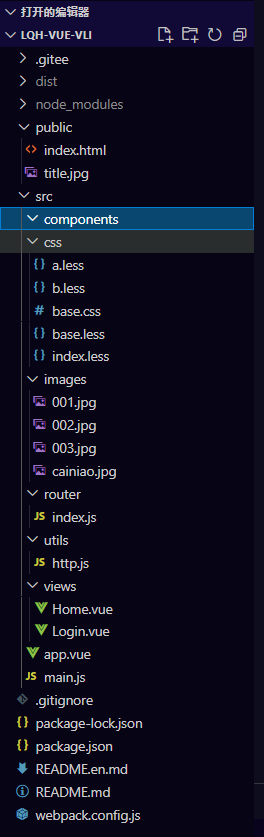技术小男生
4个月前
linux环境jdk环境变量配置
1：编辑系统配置文件vi/etc/profile2：按字母键i进入编辑模式，在最底部添加内容：JAVAHOME/opt/jdk1.8.0152CLASSPATH.:\$JAVAHOME/lib/dt.jar:\$JAVAHOME/lib/tools.jarPATH\$JAVAHOME/bin:\$PATH3：生效配置光头强的博客
4个月前
Java面向对象试题
1、请创建一个Animal动物类，要求有方法eat（）方法，方法输出一条语句“吃东西”。创建一个接口A，接口里有一个抽象方法fly（）。创建一个Bird类继承Animal类并实现接口A里的方法输出一条有语句“鸟儿飞翔”，重写eat（）方法输出一条语句“鸟儿吃虫”。在Test类中向上转型创建b对象，调用eat方法。然后向下转型调用eat（）方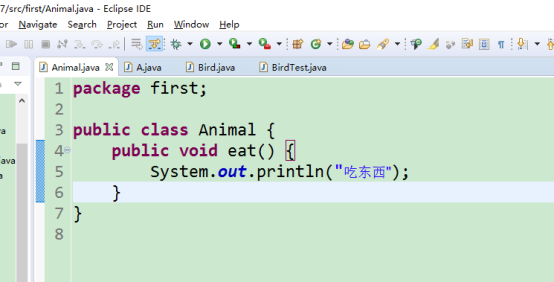刚刚好
4个月前
css问题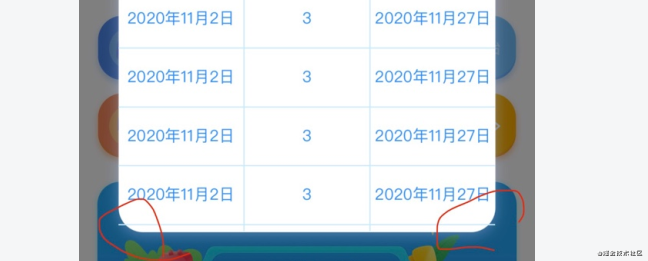blmius
1年前
MySQL:[Err] 1292 - Incorrect datetime value: ‘0000-00-00 00:00:00‘ for column ‘CREATE_TIME‘ at row 1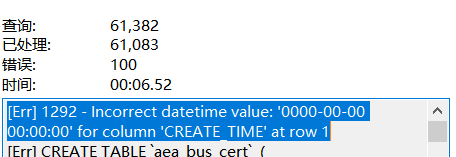小森森
4个月前晴空闲云
4个月前
css中box-sizing解放盒子实际宽高计算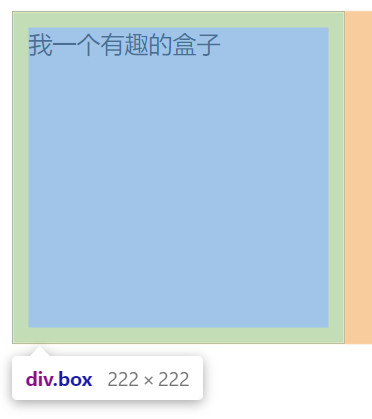Stella981
1年前
KVM调整cpu和内存Wesley13
1年前
Java集合详解1：一文读懂ArrayList,Vector与Stack使用方法和实现原理helloworld_28799839
4个月前

Javascript判断对象是否为空jsObject.keys(myObject).length0经常使用的三元运算我们经常遇到处理表格列状态字段如status的时候可以用到vueWesley13
Lv1

1.2w

9

9